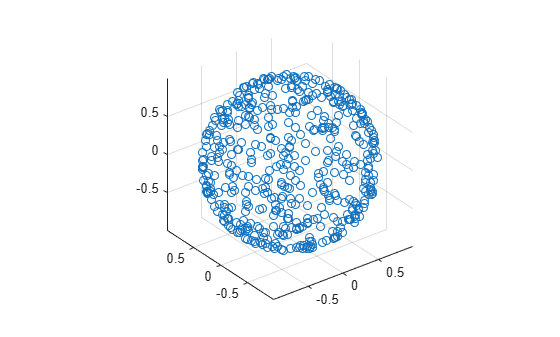# randrot

Uniformly distributed random rotations

## Syntax

``R = randrot``
``R = randrot(m)``
``R = randrot(m1,...,mN)``
``R = randrot([m1,...,mN])``

## Description

````R = randrot` returns a unit quaternion drawn from a uniform distribution of random rotations.```

example

````R = randrot(m)` returns an `m`-by-`m` matrix of unit quaternions drawn from a uniform distribution of random rotations.```

example

````R = randrot(m1,...,mN)` returns an `m1`-by-...-by-`mN` array of random unit quaternions, where `m1`,…, `mN` indicate the size of each dimension. For example, `randrot(3,4)` returns a 3-by-4 matrix of random unit quaternions.```
````R = randrot([m1,...,mN])` returns an `m1`-by-...-by-`mN` array of random unit quaternions, where `m1`,…, `mN` indicate the size of each dimension. For example, `randrot([3,4])` returns a 3-by-4 matrix of random unit quaternions.```

## Examples

collapse all

Generate a 3-by-3 matrix of uniformly distributed random rotations.

`r = randrot(3)`
```r = 3x3 quaternion array 0.17446 + 0.59506i - 0.73295j + 0.27976k 0.69704 - 0.060589i + 0.68679j - 0.19695k 0.35191 + 0.74478i + 0.52322j - 0.21842k 0.21908 - 0.89875i - 0.298j + 0.23548k -0.049744 + 0.59691i + 0.56459j + 0.56786k 0.17527 - 0.46955i + 0.52986j - 0.68414k 0.6375 + 0.49338i - 0.24049j + 0.54068k 0.2979 - 0.53568i + 0.31819j + 0.72323k -0.30189 - 0.22864i - 0.83159j + 0.40626k ```

Create a vector of 500 random quaternions. Use `rotatepoint` to visualize the distribution of the random rotations applied to point (1, 0, 0).

```q = randrot(500,1); pt = rotatepoint(q, [1 0 0]); figure scatter3(pt(:,1), pt(:,2), pt(:,3)) axis equal```## Input Arguments

collapse all

Size of square quaternion matrix, specified as an integer value. If `m` is `0` or negative, then `R` is returned as an empty matrix.

Data Types: `single` | `double` | `int8` | `int16` | `int32` | `int64` | `uint8` | `uint16` | `uint32` | `uint64`

Size of each dimension, specified as two or more integer values. If the size of any dimension is `0` or negative, then `R` is returned as an empty array.

Example: `randrot(2,3)` returns a 2-by-3 matrix of random quaternions.

Data Types: `single` | `double` | `int8` | `int16` | `int32` | `int64` | `uint8` | `uint16` | `uint32` | `uint64`

Vector of size of each dimension, specified as a row vector of two or more integer values. If the size of any dimension is `0` or negative, then `R` is returned as an empty array.

Example: `randrot([2,3])` returns a 2-by-3 matrix of random quaternions.

Data Types: `single` | `double` | `int8` | `int16` | `int32` | `int64` | `uint8` | `uint16` | `uint32` | `uint64`

## Output Arguments

collapse all

Random quaternions, returned as a quaternion or array of quaternions.

Data Types: `quaternion`

 Shoemake, K. "Uniform Random Rotations." Graphics Gems III (K. David, ed.). New York: Academic Press, 1992.